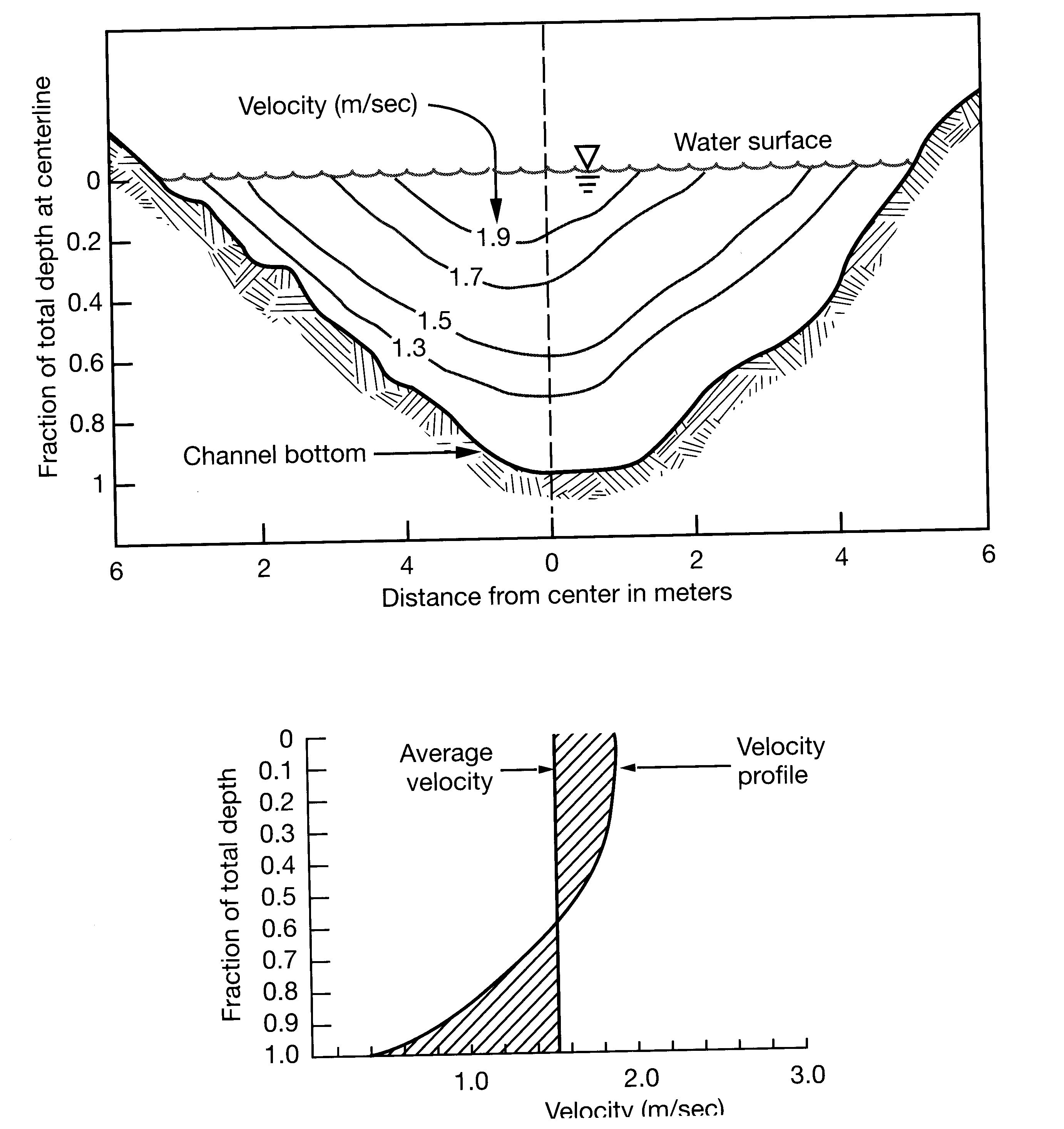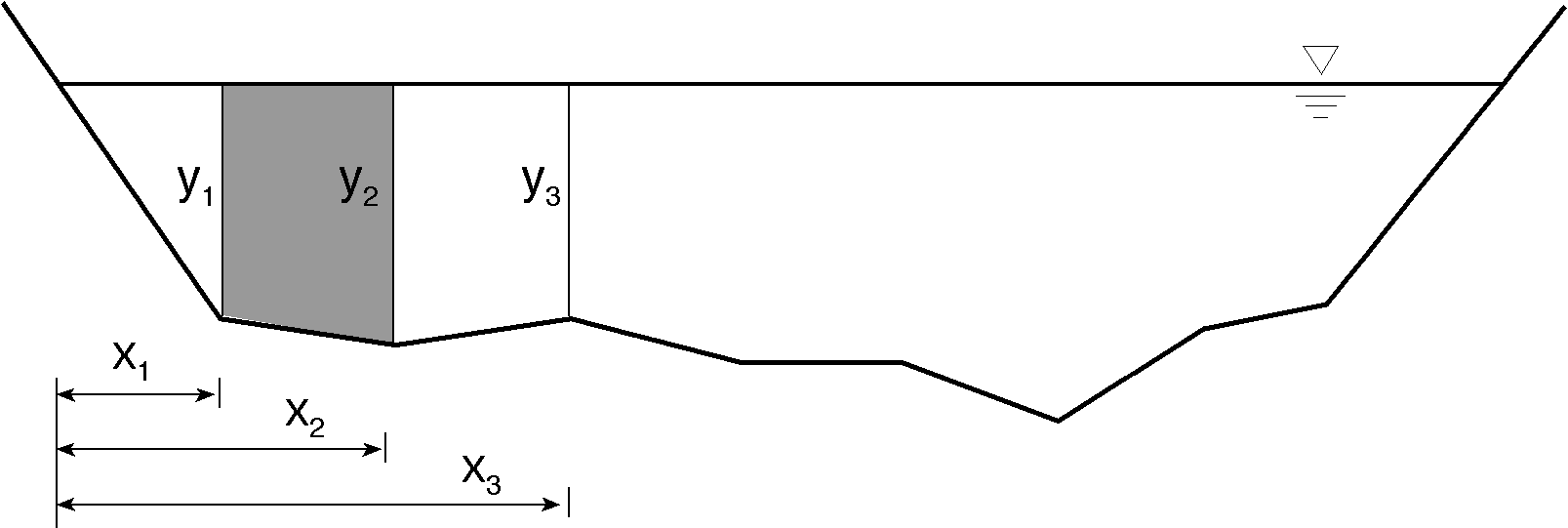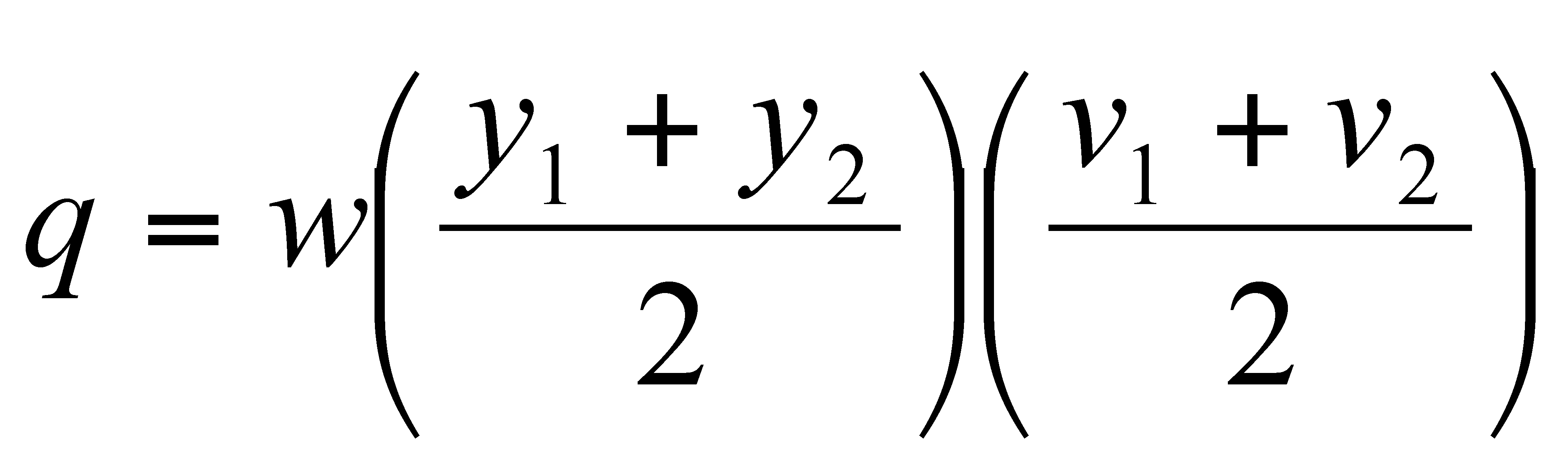## Stream Discharge Measurement : Float and Velocity-Area Methods

 Section Lab Assigned Due 012,013 Tuesday Tuesday, Sep 18 Friday, Sep 21 011 Thursday Thursday, Sep 20 Monday, Sep 24

• 40 possible points.
• WRITE NEATLY and LEGIBLY.

### PURPOSE

The purpose of this lab is for you to get hands-on experience with stream gauging by calculating the discharge of Boulder Creek using a variety of methods.  Getting outside is nice too?
Note: Some of you will need to wade through the creek. Be prepared.

### BACKGROUND

The process of measuring stream flow (volume rate of flow), or discharge, is called stream gauging.  There are numerous methods of stream gauging, including direct methods, such as volumetric gauging, and dilution methods, as well as indirect methods involving stage-discharge relations, or rating curves.  Since the velocity of a stream varies with depth and width across a stream, it is important to understand what it is you want to measure when choosing a stream gauging method.  If you are interested in stream surface velocity, the float method would work well.  This method involves throwing some buoyant, highly visible object into the stream and measuring the time it takes to float a known distance.  If you are interested in obtaining a more accurate stream discharge measurement, the velocity-area method might be your method of choice.

``
Figure 1a and 1b showing stream velocity distribution. Figure 1a is a cross -sectional view with contours indicating how velocity varies from top to bottom and across the stream channel. Figure 1b is an example of a velocity profile. Notice how how velocity changes with increasing depth, reaching the average velocity at approximately 0.6 of the total depth (or 0.4 of the depth from the bottom).

Figure 1a is an example of how the velocity of a stream can vary in the cross-stream direction and with depth.  Stream velocity is typically faster at the surface and toward the middle of the channel, and slower along the sides and bottom of the channel due to differences in friction.  The velocity profile in Figure 1b shows how the average velocity is usually at 0.6 times the total depth from the water surface, or 0.4 times the total depth from the bottom of the channel.  This is why, in shallow channels (< 2.5 ft or < 0.75 m), current meter measurements are made at four tenths of  total depth (from the bottom).  In larger streams two velocity measurements are made at each vertical and averaged; one at two tenths of the total depth and one at eight tenths of the total depth.  From these diagrams you can see why the float method could give velocities that are higher than the average stream velocity.  You can also see how the volume-area method, which involves more detailed measurements of the velocity distribution, could give a more accurate representation of the discharge.

Today you will be using two methods to calculate the discharge of Boulder Creek; the float method and the velocity-area method.  You will be measuring out a length of river for the simple float method.  For the velocity-area method you will establish a cross section through Boulder Creek and measure velocity at points along this cross section at known intervals.  Functionally, you will do this by dividing your stream into discrete sections where you can calculate the cross-sectional area and measure an average velocity (area x velocity = discharge) (Figure 2).  Then you will sum the discharges, Q, of each section to determine the total Q of the stream at that cross-section. Obviously, the more sections you include, the more accurate your determination of discharge is, but there must be a balance between accuracy and efficiency.Figure 2 An example of the velocity-area stream gauging cross-sectional set up, where x is the distance from the initial point to a vertical, and y is the depth of a vertical.Equation1 To calculate the discharge of each section, where q is the discharge of each section, w is the width of the section, y is the depth of each vertical, and v is the velocity at each vertical.Equation 2 To calculate the stream discharge (Q) of Boulder Creek you need to sum all the section discharge (q).

### CHOOSING A SITE

Before getting started making velocity measurements, you need to choose a location for your stream gauging effort.  This location depends on your question; what are you trying to measure?  Do you want to know the discharge of a stream, or the outflow from some water treatment facility?  Once you have decided what your general objective is, the site you choose should fit the following criteria:
• No eddies (or few eddies).
• A smooth cross section with minimal flow obstruction.
• Velocities and depths that do not exceed the range for which your equipment can accurately measure.
• Converging flow, or a location where the channel is not getting wider immediately down stream of your gauging location.
• ### MEASURING VELOCITY: THE FLOAT METHOD

You need:
• marking tape, red or orange
• tape measure
• stop-watch
• at least three highly visible buoyant objects such as oranges
• three people (two will work); one at the top of your reach, one at the bottom, and someone to record data
Experiment:

For the float method, measure out some convenient distance along the stream bank (try at least 30 meters).  In hydrology, this is called a "reach". Station one person at the upstream end of your selected reach and one at the downstream end.  The person at the upstream end has the stop-watch and the oranges.  The person at the top releases an orange and starts the clock when the orange floats over the top boundary of your reach.  When the orange passes the bottom boundary of your reach, the person at the bottom signals to the top person to stop the clock.  Someone records the time and notes the distance traveled.  Do this at least three times.

Calculations:

Surface Velocity = Distance / Time
Average Surface Velocity = Sum Surface Velocitites / Number of Trials

### MEASURING VELOCITY: THE VELOCITY_AREA METHOD

You need:
• tape measure
• stop-watch
• current meter
• at least two people; one to count clicks, and one to record data
Experiment:

You will be using currant meters like the Price AA or its little brother, the Pygmy to measure velocity.  We will go over the care and feeding of these meters in lab.  The measurements you make of velocity will be used to estimate the average velocity for the area, or vertical where the velocity measurement was made.  Find a place along Boulder Creek that fits the above requirements.  String a measuring tape across the channel perpendicular to the flow and secure both ends.  For this exercise you should have at least 10 verticals (mark 10 spots on the line with red tape).  It is most convenient if the spacing between the verticals is even, but it is OK if they are not.  Measure the velocity at each vertical.  You will be counting the number of clicks that occur in at least 40 seconds.  For the Pygmy meter and one of the settings on the Price AA, one click indicates one revolution of the cups. For depths of 0.75 meters (2.5 feet) or less, we assume that the average velocity can be estimated by measuring the velocity at 0.4 of the depth off the bottom (i.e. for a depth of 1 meter you would measure the velocity at a depth of 0.4 meters).  The velocity meters are mounted on wading rods that have increments of measurement on them.  You can use the rod to measure the depth at your vertical and then slide one portion of the rod such that the meter is at the desired depth of 0.4 times the depth.  After a little practice, using these rods becomes much easier than it may seem at first!

NOTE:  THESE METERS ARE DELICATE AND EXPENSIVE!  PLEASE USE CARE!  For example:  DO NOT SMASH THEM AGAINST THE ROCKS!

Keep good notes and record the distance from the bank (location of each vertical), number of clicks, time, and depth for each vertical on your data sheet.  When you have made velocity measurements for all the verticals along your cross section calculate the discharge of Boulder Creek.  To do this use the preceding equations, Figure 2, and the data you recorded in your data table.

1. SITE MAP
• Draw a map of the research site on the back side of your data sheet.
• Draw a cross-section of the area that we are profiling on the back side of your data sheet.  Label each section where discharge will be measured.

2. MEASUREMENTS
In the field, record the information necessary to calculate the discharge using:
• float method
• velocity-area method

3. CALCULATIONS
For the float method:
• What is your average velocity from the float method?
• What is the standard deviation of your three surface velocity measurements?
• What is your discharge value in cfs for the float method? in m3/s?                                          ,

For the velocity-area method:
• In EXCEL, make a table similar to your handout that allows you to calculate discharge. Print out your excel spreadsheet.
• What is your discharge value in cfs from the velocity-area method?
• What is your discharge value in m3/s from the velocity-area method?

4. COMPARE THE TWO METHODS
• Which discharge value is higher?
• Why? Use a minimum of 5 sentences and a maximum of one page to answer this question.

5. COMPARE YOUR VALUE WITH USGS PUBLISHED DATA
• Go to the USGS web site:  http://waterdata.usgs.gov/co/nwis/current/?type=flow&group_key=huc_cd
• Print out the discharge for the Boulder Creek site at 75th street for the day of your measurements.  Include this graph with your lab assignment.
• Interpolate from that graph and find the discharge in cfs at noon of the day of your measurements?  in m3/s?                                         ,
• Compare your two discharge measurements with the USGS value. Why might they be similar or different?